Build Deep Learning model for the Image Classification task (Part1): Pytorch model

Phong Le

# Build Deep Learning model for the Image Classification task (Part1): Pytorch model

The AI, Machine Learning, and Deep Learning definition are becoming viral recently. Let's see how we could apply them to address our problems in the real world, especially in the Computer Vision field for the Image Classification task.As you know AI or Machine Learning/ Deep Learning has gained a reputation recently. In the real world, we can apply the AI solution to a lot of data types like image, text, and excel… data and today I will introduce you to Computer Vision techniques and how to build some deep learning models for the important task in the Computer vision field, Image classification task. The image classification task is that you want the machine to automatically classify the natural image in a specific category, for example, if the input image consists of a dog object we could classify it as a dog image.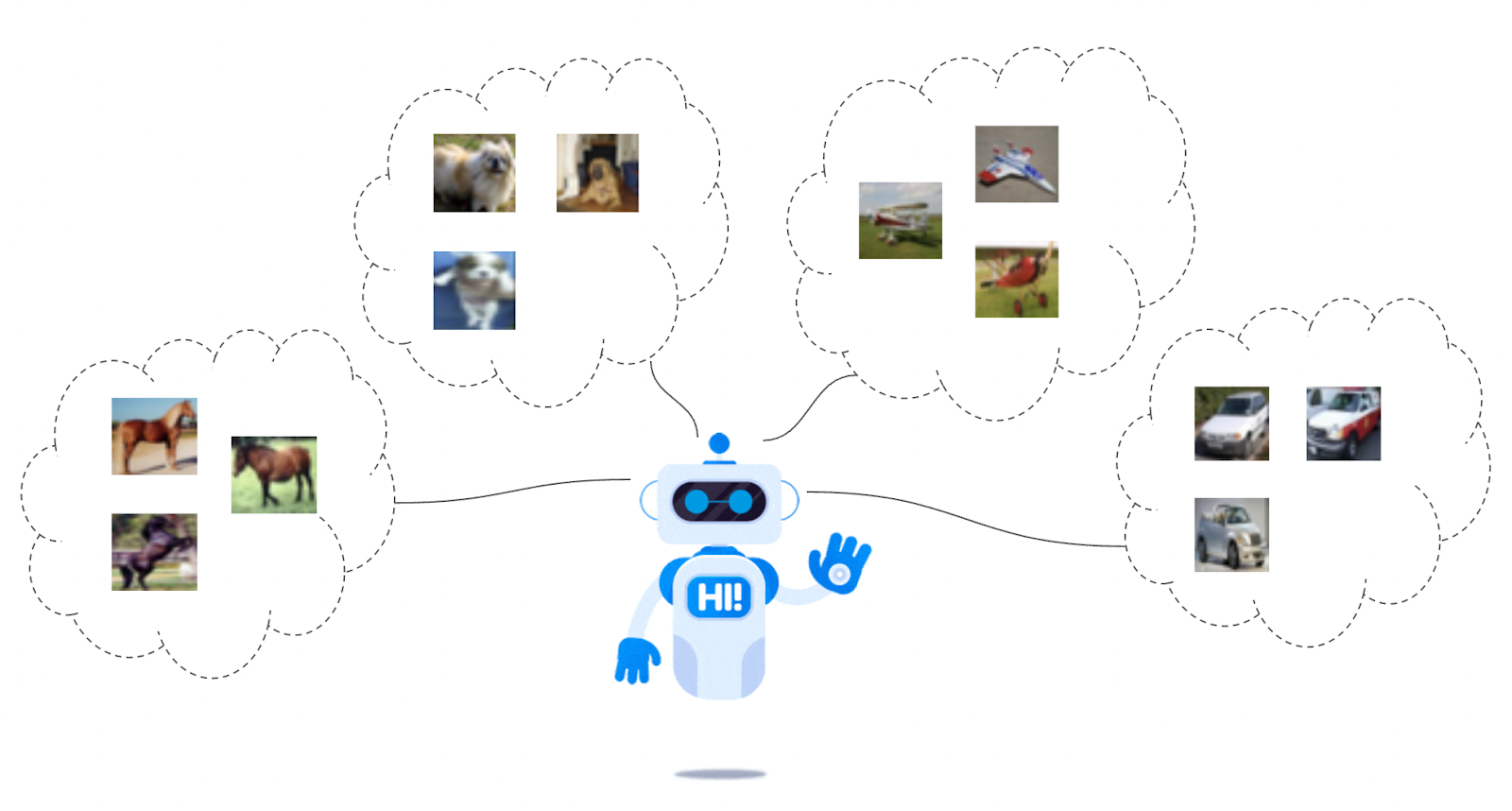In this article, I want to show you the overall Computer Vision techniques, and I will not focus on the detailed algorithm or formula of each step. So this article might need you to understand a little bit about the AI/ Machine Learning or Deep Learning definition or their related knowledge, but don’t worry I will try to describe all of them simply and make sure you could understand to run my example each line by line code, I hope you could use them on your dataset or apply them to address your specific issues.

• The first section is about the Dataset, the data preparation step, and the data folder structure
• In the second section, I will explain my implementation to build the Image Classification model and some related modules.
• The final section is the instruction section for you to easily use my source code on your dataset. If you do not want to go into detail or it’s difficult to understand all of them, feel free to jump into the final section for applying the source code in your project.

## 1) Data

Firstly, I want to share with you how the dataset preparation is. I will not make it so difficult for you to prepare your dataset. To classify all images into each label folder, we need to prepare the training dataset into each class folder, for example, we want to classify three types of images: person, cat, and dog. We will create three folders including these sample images for a person, cat, and dog. In this article, I will use a popular dataset for image classification tasks, the CIFAR10 dataset.

The CIFAR-10 dataset has 60000 of 32x32 color images in 10 classes, with 6000 images per class. This dataset has about 50000 images for the train set and 10000 images for the test set. There are ten classes in the CIFAR-10 dataset: airplane, automobile, bird, cat, deer, dog, frog, horse, ship, and truck.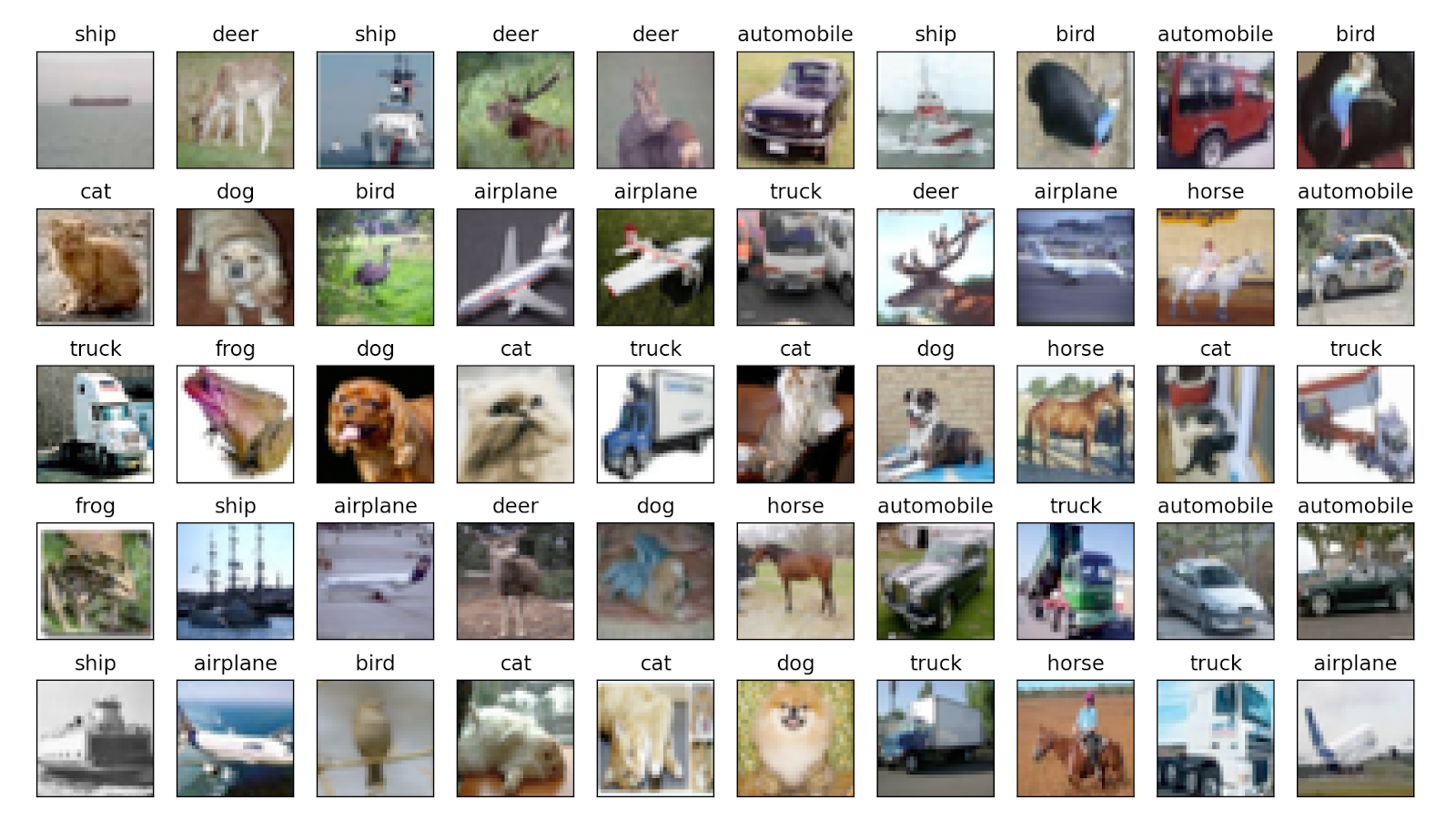Figure2: CIFAR-10 Dataset

For the train folder, we will create like the below, in the `data/train` path, and we have 10 folders to store the sample images for each class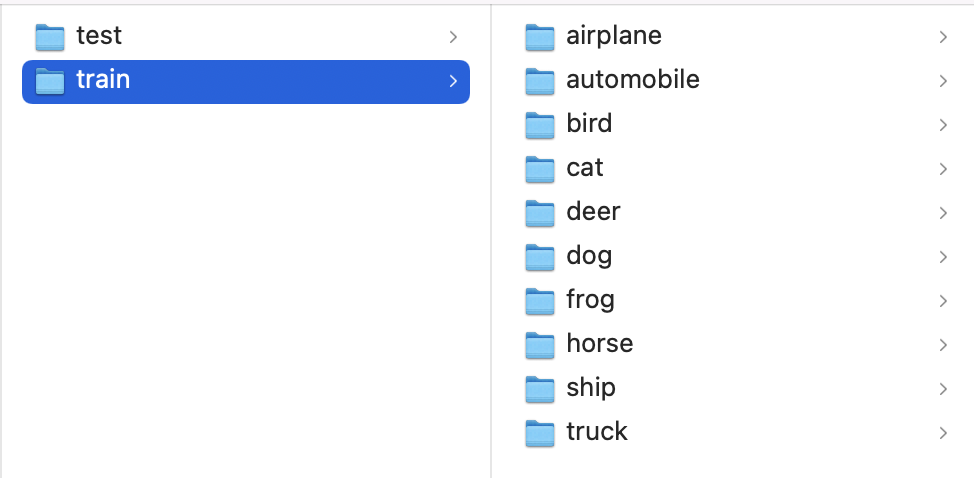Figure3: Train folder structure

The test folder is also the same as the train folder, we also have 10 folders for 10 classes but the image and the number of images will be different from the train folder.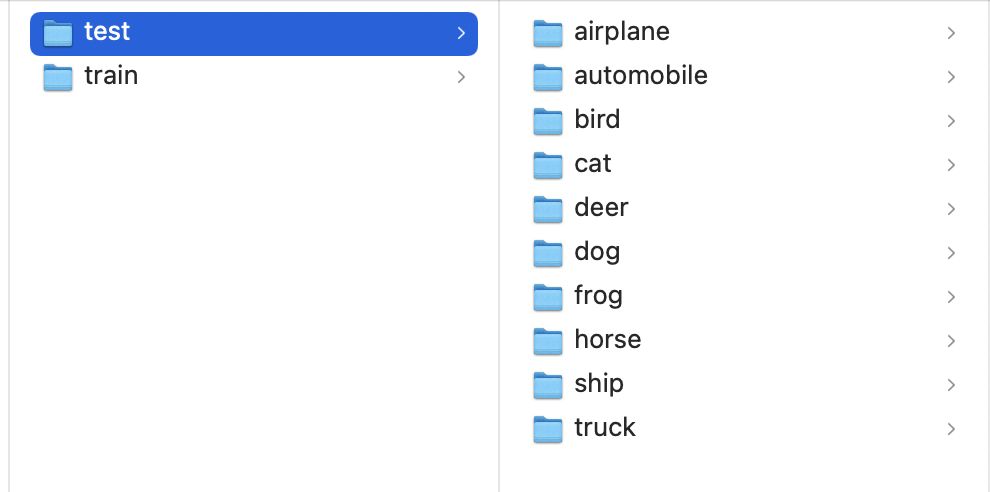Figure3: Test folder structure

## 2) Implementation

In this section, I will insulate for you the whole basic pipeline for building Deep Learning models for the Image Classification task. It will be separated into some sections as below:

• Define training hyperparameters
• Create Torch Dataset and Data Loader
• Create Model
• Define metrics
• Create training process
• Create evaluation process

### 2.1) Define training hyperparameters

Firstly, we will define some argument parameters for the training processing, in Python, we will create them as some variables. I usually put all of them in one section area in the code to easily change the programming process. It will be very easy for us to change the program running via these parameters

# Hyper parameters

batch_size = 32

h, w, c = 32, 32, 3

num_class = 10

epochs = 20

learning_rate = 0.01

train_data_path = 'data/train'

test_data_path = 'data/test'

result_dir = 'model/cnn_model.pth'

• `batch_size`: when training a Convolutional Model or Deep Learning model, to save training time for processing a batch of data we will use this param to set up the number of images for one forward processing
• `h, w, c`: these parameters will be the height, width and channel of the input image. For the CIFAR10 Dataset, each image will have a 32x32x3 image size so we are setting h, w, and c are 32, 32, and 3. It’s also an input size for your Deep Learning model, if these parameters are changed the model size will be changed together but the architecture will be kept the same.
• `epochs`: we will use this parameter to specify the number of repeats for the training process, e.g if epochs=20, we will run the training processing 20 times for optimizing the model
• `learning_rate` will be used for the learning process.
• `train_data_path`, `test_data_path`, `result_dir`: they will be used to specify the folder path for each step in the training process

You could try to change these parameters here to adjust your own application.

### 2.2) Create Torch Dataset and Data Loader

This is a very important step, we need to manage the read/write and process dataset in this step effectively. The output of this step will be used for the training model, so if it has any issues will also affect the model performance results

Because we are using the Pytorch framework for building our CNN model, we need to define the corresponding data format for the Pytorch model too. Another reason is that the dataset processing sometimes can get messy and hard to maintain, so ideally we will use 2 Pytorch official classes for defining the dataset: torch.utils.data.Dataset and torch.utils.data.DataLoader.

The Dataset object will be used for some pre-loaded datasets as well, it will store the data sample for training and their corresponding ground truth or labels. There are three main methods that we need to define in the Dataset object: __init__(), __getitem__(), and __len__()

• __init__(): in this method, we need to set up some important attributes for our Dataset object which are used throughout the Dataset object. For example, I created the CIFAR10Dataset() object to get the given input (data_path, transform methods) and initialize the self.classes for mapping from id number to label text, or self.label_id for mapping from label text to id number, the self.data_path and self.transform will be reused throughout this object, the self.image_names will be used to store all image paths in the CIFAR10 folder and the self.labels will store the corresponding label for each image path in integer number
•

class ImageDataset(Dataset):

"""

"""

def __init__(self,

data_path: str="data/train",

transform: transforms=None

):

self.classes = {i: v for i, v in enumerate(os.listdir(data_path))}

self.label_id = {v: i for i, v in enumerate(os.listdir(data_path))}

self.data_path = Path(data_path)

self.transform = transform

"""

"""

self.image_names = []

self.labels = []

for _data in os.listdir(self.data_path):

sub_data = [f"{self.data_path}/{_data}/{file_name}" for file_name in os.listdir(self.data_path / _data)]

self.image_names = self.image_names + sub_data

self.labels = self.labels + [self.label_id[_data]] * len(sub_data)

• __getitem__(): in this method, we will have the default given input for the index, and we need to return the specific data sample or image for this index with the corresponding label for a data sample, these data will be used for each step training later, so we could write the process like this

def __getitem__(self, index: int):

image_path = self.image_names[index]

label = self.labels[index]

img = Image.open(image_path)

if self.transform is not None:

img = self.transform(img)

label = torch.tensor(label)

return img, label

• __len__(): finally the __len__() method will return the total number of data for our training or testing dataset, so we just need to return the length of data like this

def __len__(self):

return len(self.labels)

So we will have 2 training set objects and testing set objects as below

train_dataset = ImageDataset(

data_path=train_data_path,

transform=train_tranforms

)

test_dataset = ImageDataset(

data_path=test_data_path,

transform=test_tranforms

)

About `train_transforms` and `test_transforms` definitions, they are just built-in processing steps of Pytorch, and we could apply them for each data sample for converting format, resizing tensor, normalizing sample, or augmentation tasks…

import torchvision.transforms as transforms

train_tranforms = transforms.Compose([

transforms.Resize(h),

transforms.ToTensor(),

transforms.Normalize(mean=mean, std=std)

])

test_tranforms = transforms.Compose([

transforms.Resize(h),

transforms.ToTensor(),

transforms.Normalize(mean=mean, std=std)

])

After having the Pytorch Dataset Object for the CIFAR10 dataset, we will continue creating the DataLoader Object for the training step. The Pytorch DataLoader is something that could wrap an iterable around the previous Dataset Object to enable easy access to the data samples. For simple understanding, the DataLoader is just an iterable object and we could go through all Dataset by using it easily.

In this DataLoader object, we could see there are two main parameters: batch_size is used for specifying the number of data samples that will be processed once training time, and the shuffle flag will be used to define if we need to shuffle all data or not

We could test the Dataset and DataLoader objects by using the code below:

# Obtain one batch of data samples

images, labels = next(dataiter)

# Convert images to numpy for display

images = images.numpy()

print(images.shape, labels)

### 2.3) Create Model

In this section, I will show you how to build some basic Model architecture for the Image classification task. Here we have 2 examples of Convolutional models for the Image Classification task: the basic CNN model and the Pre-trained Deep Learning model from the Pytorch hub

#### 2.3.1) CNN model

For you to understand how to build a Deep Learning model from the basics using the Pytorch framework, I have built a CNN model from scratch. This model will include three Convolutional Blocks and 2 hidden fully connected layers.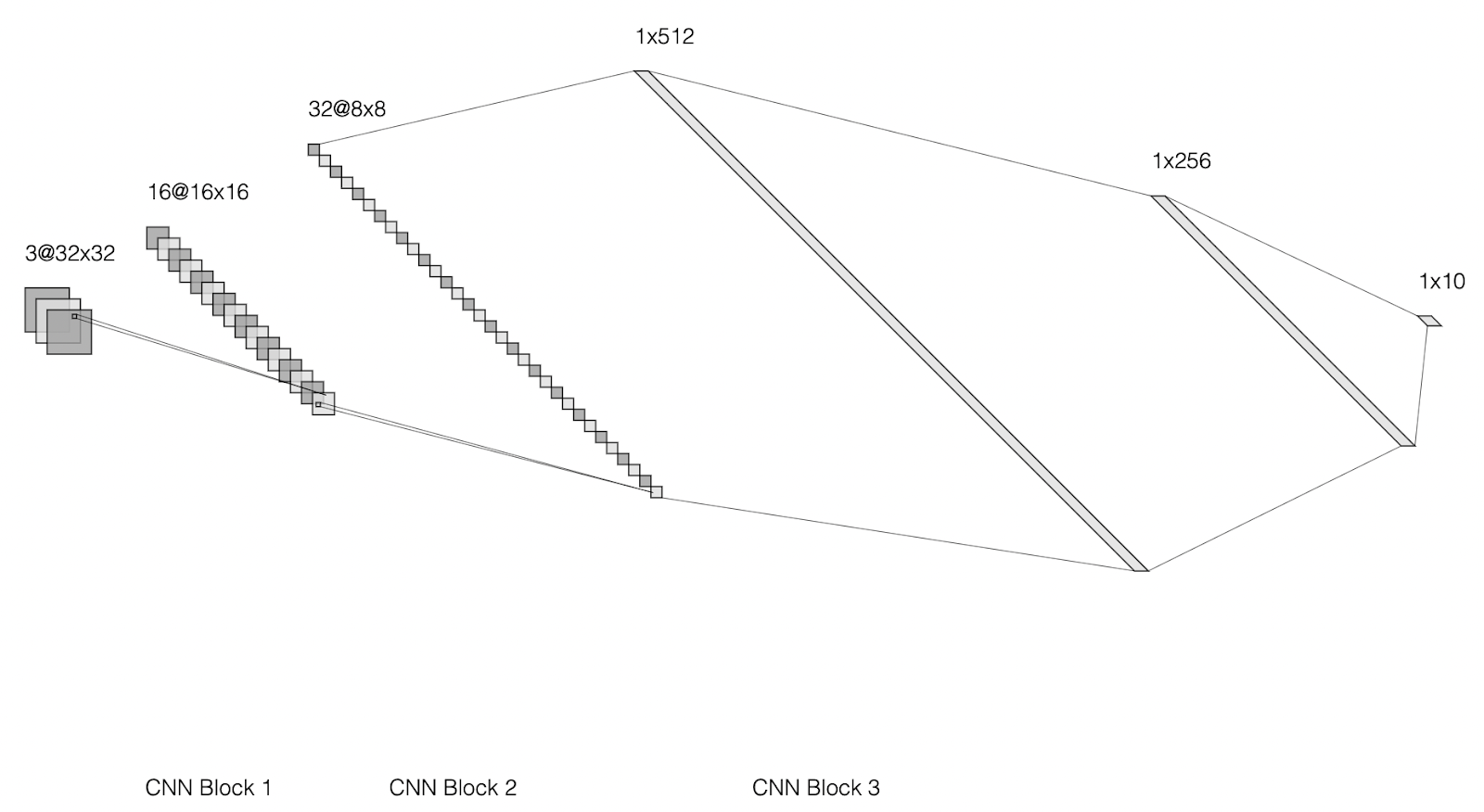Figure4: CNN model architecture

You could see the model architecture in the Figure4, we have some states as below

• Input: have 32x32x3 size for a loaded image file from the `data` folder
• CNN Block1:  one Convolutional layer (input_dim= 32x32x3, kernel_size= 16x3x3, stride_window=1, padding =1), ReLu activation function, and the Max Pooling Layer (kernel_size=2x2) => output_dim = 16x16x16
• CNN Block2:  one Convolutional layer (input_dim= 16x16x16, kernel_size= 32x3x3, stride_window=1, padding =1), ReLu activation function, and the Max Pooling Layer (kernel_size=2x2) => output_dim = 32x8x8
• CNN Block3:  one Convolutional layer (input_dim= 32x8x8, kernel_size= 64x3x3, stride_window=1, padding =1), ReLu activation function, and the Max Pooling Layer (kernel_size=2x2) => output_dim = 64x4x4
• Flatten Layer: the input tensor size 64x4x4 will be flattened into 64*4*4=1024 vector
• Dropout Layer will be used to drop some random node in the Fully connected layers for avoiding the bias or overfitting problem
• Fully Connected Layers: we have 2 hidden layers: the first layer has 1024x500 weight size and the second layer has 500x10 weight size. And the output of the second fully connected layer will be the output of the whole CNN model for ten classes respectively.

With the Pytorch framework, it provides us with all the necessary libraries for building Convolutional, MaxPool, Dropout, or Fully Connected layers, and we will wrap all of them into one CNNModel() class using nn.Module of Pytorch.

import torch.nn as nn

import torch.nn.functional as F

# define the CNN architecture

class CNNModel(nn.Module):

def __init__(self, num_class:int=10):

super().__init__()

# Convolutional layer (sees 32x32x3 image tensor)

self.conv1_layer = nn.Conv2d(in_channels=3, out_channels=16, kernel_size=3, stride=1, padding=1)

# Convolutional layer (sees 16x16x16 tensor)

self.conv2_layer = nn.Conv2d(in_channels=16, out_channels=32, kernel_size=3, stride=1, padding=1)

# Convolutional layer (sees 8x8x32 tensor)

self.conv3_layer = nn.Conv2d(in_channels=32, out_channels=64, kernel_size=3, padding=1)

# Max pooling layer

self.pool_layer = nn.MaxPool2d(kernel_size=2, stride=2)

# Linear layer (64 * 4 * 4 -> 500)

self.fc1_layer = nn.Linear(in_features=64 * 4 * 4, out_features=500)

# Linear layer (500 -> 10)

self.fc2_layer = nn.Linear(in_features=500, out_features=num_class)

# Dropout layer (p=0.25)

self.dropout_layer = nn.Dropout(p=0.25)

def forward(self, x):

# CNN Block 1

x = self.conv1_layer(x)

x = F.relu(x)

x = self.pool_layer(x)

# CNN Block 2

x = self.conv2_layer(x)

x = F.relu(x)

x = self.pool_layer(x)

# CNN Block 3

x = self.conv3_layer(x)

x = F.relu(x)

x = self.pool_layer(x)

# Flatten layer

x = x.view(-1, 64 * 4 * 4)

# Dropout layer

x = self.dropout_layer(x)

# Full connected block: 1st hidden layer

x = self.fc1_layer(x)

x = F.relu(x)

x = self.dropout_layer(x)

# Full connected block: 2nd hidden layer

x = self.fc2_layer(x)

return x

After defining the model architecture, we could call and create the CNN model instance by these commands as below:

# Define model

device = torch.device("cuda" if torch.cuda.is_available() else "cpu")

# device = "cpu"

model = CNNModel(num_class)

model = model.to(device)

• CNN model: We will build some basic layers of Convulional block from scratch, and use them to train from scratch on our dataset or CIFAR10 dataset

#### 2.1.2) Pretrained model

For the Pretrained Model (VGG16): We will try a very popular pre-trained model from PyTorch model zoo. Pytorch also provides us with some available pre-trained models that we could call and use easily. So What is the pre-trained model? There are some available that were trained before on very general or large datasets like ImageNet, COCO, VOC… It means these models are very good for image processing or feature extraction tasks with high performance and we could reuse them for our task, just need to retrain on a few datasets and it also takes no more time for retraining the whole model architecture. In this example, I will choose the VGG16 model, a very popular model which was trained on the ImageNet dataset before for the image classification task too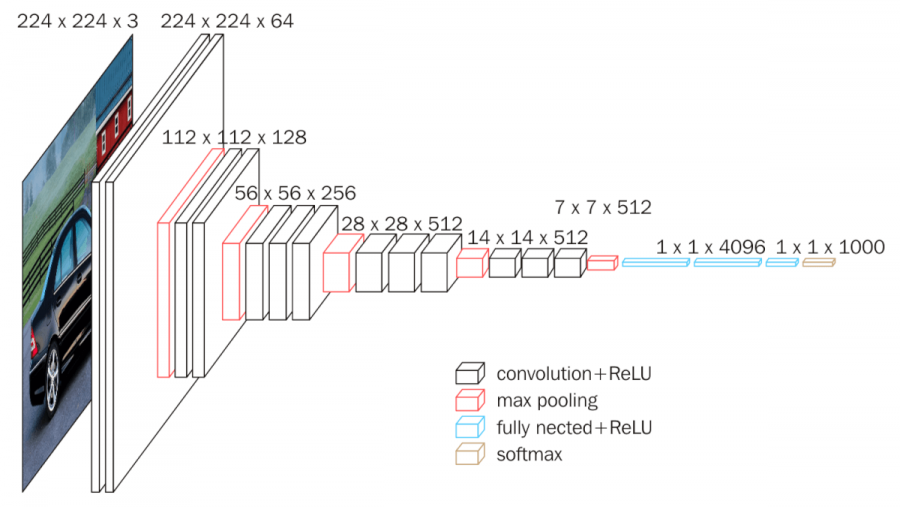Figure5: VGG16 model architecture (image from https://paperswithcode.com/method/vgg )

In order to reuse this model in the Pytorch, we just need a few codes as below:

model = models.vgg16(pretrained=True)

fc_features = model.fc.in_features

model.fc = nn.Linear(fc_features, 10)

### 2.4) Define metrics

In this step, we will define the loss function for our model as well as the optimizer algorithm for the model learning process to update the weight parameters

# computes softmax and then the cross entropy

criterion = nn.CrossEntropyLoss()

### 2.5) Create training process

The main idea of the training process is to go over all datasets (in the previous DataLoader), and at each data sample (x value) we will compute the output of the model (logit value), compare with the ground truth label (y value) to compute the loss value, and then use this loss value for the defined optimizer algorithm above to update the weights for model.

We will continually repeat this process for epoch number time until the loss value is small enough to get the best-optimized model (loss value should be smaller than a defined threshold)

# Training

for epoch in range(epochs):

train_sum_loss = 0

train_sum_acc = 0

test_sum_loss = 0

test_sum_acc = 0

model.train()

x = x.to(device)

y = y.to(device)

# Compute output

logit = model(x)

loss = criterion(logit, y)

loss.backward()

# Backpropagation

optimizer.step()

train_sum_loss += loss.item()

_, pred = torch.max(logit, 1)

train_sum_acc += (pred==y).float().mean()

### 2.6) Create evaluation process

Each epoch, we will also evaluate the updated model on the test set again to see if our trained model working fine on the test set or not

The evaluation process is also the same as the training process, we also need to go over all the test set and compute the output of the model (logit value) with the given input (x_test value), then we also compute the loss value and the accuracy value as well on the test set.

# Testing on test set

model.eval()

x_test = x_test.to(device)

y_test = y_test.to(device)

logit = model(x_test)

loss = criterion(logit, y_test)

test_sum_loss += loss.item()

_, pred = torch.max(logit, 1)

test_sum_acc += (pred==y_test).float().mean()

print('Epoch {}: Train loss: {} -- Test loss: {} -- Train Acc: {} -- Test Acc: {}'.format(

))

Finally after having the well-trained model, we could store it as a .pth file to could easily load it for use in the next time and no need to retrain the whole model again

# Saving model

torch.save(model.state_dict(), result_dir)

## 3) Compare training results

In this section, I will summarize the training results of two experiments: train CNN model from scratch and train VGG16 model based on pre-trained from ImageNet Dataset.  Figure6: Training result of CNN model on CIFAR10

Figure7: Training result of VGG16 model on CIFAR10

As you could see, the performance of VGG16 model is higher than that of CNN model, it might be that the VGG16 model is larger than CNN model and we are using the pre-trained weight from ImageNet Dataset and it provides us a good Convolutional feature extraction layers.

## 4) Train on your dataset

If it’s hard for you to understand the whole steps above, please don’t worry, you could focus on this section first for trying some toy examples on your own dataset, it’s very easy, you want to classify the image in the specific category, so you just need to prepare the dataset and run my script to train and export into the model file, and then you could use the output model file for testing classification on your image.

For example, if you want to classify one image as about the dog or cat, you will need to prepare some image samples in the data/train/dog folder and data/train/cat folder. Then, just need to run my script and the task will be done

Resources2nd & 3rd Floor, M.I.D Building, 02 Nguyen The Loc Street, Ward 12 Tan Binh District, Ho Chi Minh City, Vietnam

Aloha OfficeDa Nang OfficeSingaporeOpening Jobs

Life at saigontechnology

Blog